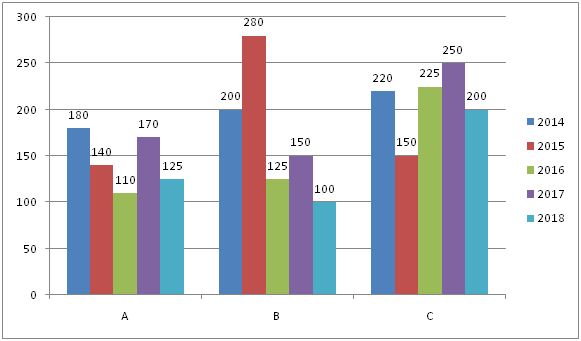# IBPS RRB PO Prelims Quantitative Aptitude Questions 2019 (Day-09)

Dear Aspirants, Our IBPS Guide team is providing new series of Quantitative Aptitude Questions for IBPS RRB PO Prelims 2019 so the aspirants can practice it on a daily basis. These questions are framed by our skilled experts after understanding your needs thoroughly. Aspirants can practice these new series questions daily to familiarize with the exact exam pattern and make your preparation effective.

Check here for IBPS RRB Prelims Mock Test 2019

[WpProQuiz 6675]

Directions (1 – 5): In each of these questions, a number series is given. In each series, only one number is missing. Find out the missing number.

1) 4, 6, 15, 49, 201, 1011,?

a) 6500

b) 5430

c) 2095

d) 6073

e) 4609

2) 24, 38, 66, 108, 164, 234, ?

a) 582

b) 318

c) 389

d) 289

e) 262

3) 6754, 6729, 6680, 6599, 6478, 6309, ?

a) 7935

b) 5837

c) 6084

d) 6893

e) 9642

4) 2, 18, 82, 226, 482, 882, ?

a) 1458

b) 1467

c) 1896

d) 1870

e) 6198

5) 52, 208, 1040, 6240, 43680, ?, 3144960

a) 398790

b) 386950

c) 398650

d) 349440

e) 368750

Directions (6 – 10): Study the following charts and answer the given questions.

The following bar graph shows that the number of students studying in branches A, B and C in a particular college from 2014 to 2018.6) By what percentage is the total number of students studying in branch B greater than those in branch C in the year 2015?

a) 32.67%

b) 86.67%

c) 93.67%

d) 64.67%

e) 54.67%

7) What is the ratio between the total students in branch A from 2016 to 2018 to the total students in branch C for 2014 and 2016?

a) 83: 78

b) 52: 75

c) 67: 82

d) 63: 89

e) 81: 89

8) What is the difference in percentage change in the number of students of branch A and branch C in the year 2018?

a) 8.3%

b) 6.2%

c) 7.2%

d) 6.47%

e) 2.73%

9) In the year 2019, the number of students in branches A, B and C increases by 10%, 12.5% and 15% respectively from the year 2014, what is the difference between the total number of students across branches in 2019 and 2017?

a) 179

b) 163

c) 180

d) 106

e) 633

10) What is the percentage change in the number of students of branch B for the year 2017 as compared to 2016?

a) 25%

b) 20%

c) 35%

d) 15%

e) 50%

Direction (1-5) :

4 * 1 + 2 = 6

6 * 2 + 3 = 15

15 * 3 + 4 = 49

49 * 4 + 5 = 201

201 * 5 + 6 = 1011

1011 * 6 + 7 = 6073

The pattern of the series is +14, +28, +42, +56…………

6754 – 25 = 6729

6729 – 49 = 6680

6680 – 81 = 6599

6599 – 121 = 6478

6478 – 169 = 6309

6309 – 225 = 6084

The pattern of the series is + 42, + 82, + 122, + 162, + 202, + 242

The pattern of the series is *4, *5, *6,*7……………

Direction (6-10) :

In 2015, Number of students in branch B= 280 and Number of students in branch C = 150

Hence required percentage = [(280 – 150)/150] * 100

= 86.67%

Total number of students in branch A from 2016 to 2018 = 125 + 170 + 110

= 405

Total number of students in branch C from 2014 and 2016 = 225 + 220

= 445

Required ratio = 405: 445

= 81: 89

Percentage change for branch A in the year 2018 compared to 2017

= [(170 – 125)/170] * 100

Percentage change for branch C in the year 2018 compared to 2017

= [(250 – 200)/250] * 100

= 26.47%

Required difference = 26.47 – 20 = 6.47%

Total number of students in 2019 = (110% of 180) + (112.5% of 200) + (115% of 220)

= 198 + 225 + 253

= 676

Now total students in 2017= 170 + 150 + 250

= 570

Thus required difference = 676 – 570

= 106

Number of students in branch B in the year 2017 = 150

And Number of students in branch B in the year 2016 = 125

Thus required percentage change = [(150 – 125)/125] * 100

= 20%